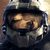0
Not a bug

dattorro 2 years ago updated by Pavel Holoborodko 2 years ago

Matlab assumes matrices to be multi-dimensional.

But Multiprecision Toolbox assumes exported matrices are only two-dimensional (can be written on a piece of paper); e.g,

Matlab input:

A = rand(3,3,4,'mp');

mp.write(A, 'mpmatrix.txt');

Matlab output:

Error using mp (line 1331)
Error: uneven number of elements in the rows.
A = mp(['[',s,']']);

This cripples communication by file transfer with Mathematica which understands Matlab .mat file format.Not a bug

@"Change .txt to .mat in above; i.e"

This changes only the extension in file name (txt to mat), but file is still textual.

That is why Mathematica can read it.

Functions mp.write and mp.read were provided solely for the purpose of transferring toolbox's arrays to other software. This can be efficiently done only in textual format. In txt format we store only 2D slices for maximum compatibility with other programs.

The MATLAB's MAT files are of binary format, it is different beast. Toolbox's multiprecision arrays can be stored in binary MAT-files and loaded by toolbox on different OS - using MATLAB's standard commands, save/load.

But Mathematica cannot read it (because there is no support for our toolbox there).Under review

Textual format is quite limited and storing multidimensional arrays in one txt file is a pain (especially in transferrable way to other software). So this is not really a bug, this is limits of txt.

The quick workaround is to store 2D slices of multidimensional array in different txt files. And then load and assemble slices back into multidimensional array in Mathematica.

For example, you need to store 4 slices of A: A(:,:,1), A(:,:,2), A(:,:,3), A(:,:,4) and then merge them back after reading from files.Change .txt to .mat in above; i.e,

Matlab input:

A = rand(3,3,4,'mp');

mp.write(A, 'mpmatrix.mat');

Matlab output:

Error using mp (line 1331)
Error: uneven number of elements in the rows.
A = mp(['[',s,']']);

This .mat file format is read/writable to both Mathematica and Matlab.

But it coughs on import of multidimensional arrays.  Should it not, if not textual?

This makes communication inelegant, by file transfer with Mathematica.